# Tensors, matrices, and MATLAB

A common source of confusion and error when dealing with different representations of rotations is to inadvertently juxtapose matrix and tensor notations. The resulting error can be further compounded when implementing rotations in computing environments such as MATLAB or Mathematica. Here, we attempt to clear up the source of this confusion. Our main point of emphasis is as follows. Given a rotation, we can use a matrix representation and express the rotation in terms of a matrix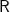. Alternatively, we can represent the rotation using a rotation tensor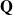. We show that the components of these two quantities are related by

(1)We also address this correspondence in MATLAB implementations of rotations and discuss compound rotations. Because matrix representations of rotations commonly feature in computer codes, our remarks pertaining to compound rotations are also relevant to codes.

Contents

## Rotation tensors and their equivalent rotation matrices

Consider a counterclockwise rotation through an angle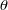about an axisthat transforms the set of right-handed basis vectors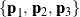into a new set of basis vectors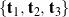. The transformation between the two bases is achieved by a rotation matrixand can be expressed in the following manners:

(2)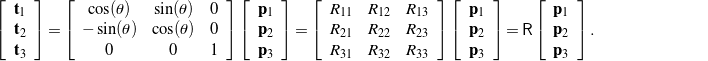Notice that ifis negative, then the direction of the rotation becomes clockwise. The corresponding rotation matrix is the transpose of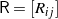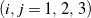, denoted by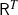. This inverse rotation is also observed when the new basisis transformed to the original basis:

(3)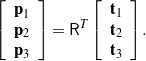Extrapolating from the above example, for a transformation corresponding to a rotation through an angleabout an axis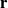, we can express the basis vectorsas a linear combination of the vectors forming the basis:

(4)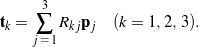It is of interest here to compare the representation (4) to the corresponding representation using a rotation tensor. To this end, we consider the same transformation fromtoexpressed using a rotation tensor:

(5)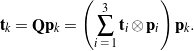The components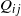of the rotation tensorare defined by the representation

(6)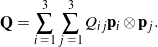How are the components of the tensorrelated to the components of the matrix? To compare and contrast (6) and (4), one can consider a vector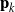acted upon by. Observing that there are two equivalent representations for the transformation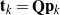,

(7)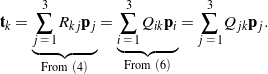As a result, we can conclude that

(8)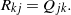An alternative method to determine how the tensor components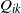relate to the matrix components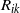is to start with (5) and (6) and then invoke (4) as follows:

(9)Note the relabeling of the indices in the final representation. Comparing the final representation to the first in (9) yields the conclusion (8). Often, the componentsof the rotation tensorare written in the matrix form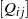. This matrix is easily confused with the rotation matrix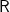. Because the rotation tensor is generally not assumed to be symmetric, this confusion will cause the direction of the rotation to be reversed.

To illustrate (8), let us return to the example of a rotation about an axisthrough an angle. Expressed as a tensor, this rotation has the representation

(10)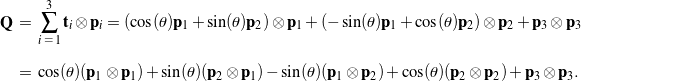The components of the rotation tensorexpressed as an arrayare readily compared to the components of the corresponding rotation matrixin (2):

(11)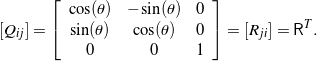As expected, the relationship between the components ofandagrees with (8).

## MATLAB implementation

One of the most convenient manners to represent vectors in MATLAB is to express them as column vectors. However, the downside of this approach is that we can only write the components of a vectorin just one basis, say,:

(12)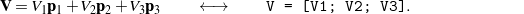Consequently, if we wish to express the rotated vector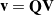as a column vector in MATLAB, we must write it in components relative to the basis. However, we can take advantage of the tensor representation

(13)When implemented as a matrix-vector operation,

(14)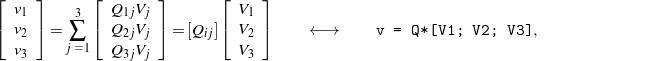where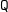is a 3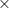3 array representing the matrixof the components of the rotation tensorsuch that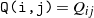. Again,should not be confused with the corresponding rotation matrix.

## Compound rotations

Consider the following sequence of rotations associated with a 3-2-1 set of Euler angles: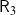through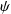about, then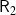throughabout, and lastly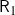through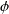about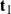. The rotation matrix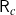that corresponds to the compound rotation is

(15)The order of multiplication for the three matrices is important to note. We know from (8) that the arrayof the components of the associated rotation tensoris related to the rotation matrixas follows:

(16)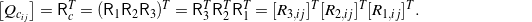Explicitly,

(17)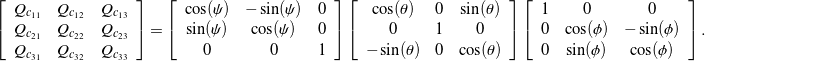Thus, the compound rotation tensorcan be represented in MATLAB as a 33 arraypopulated with the tensor componentssuch that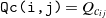, whereis easily constructed by the matrix multiplication in (17).